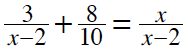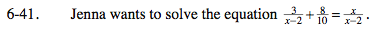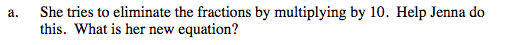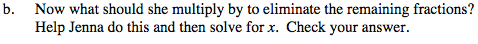### Home > INT3 > Chapter 6 > Lesson 6.2.1 > Problem6-41

6-41.
1. Jenna wants to solve the equation. Homework Help ✎

1. She tries to eliminate the fractions by multiplying by 10. Help Jenna do this. What is her new equation?

2. Now what should she multiply by to eliminate the remaining fractions? Help Jenna do this and then solve for x. Check your answer.Multiply each term by 10.

$\frac{30}{x-2}+8=\frac{10x}{x-2}$What denominator remains?

She should multiply by (x − 2). x = 7